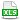HomeTechniques and Tips@RISK Simulation: Graphical ResultsInterpreting Scenario Graphs

# 7.18. Interpreting Scenario Graphs

Applies to:
@RISK 6.x/7.x

I clicked the "%" icon in Browse Results and selected a scenario. How do I interpret the numbers in the bars?

Let's work with this graph, which was produced by the first scenario in the attached workbook. (The workbook has a fixed random number seed, so that you can run a simulation and get the same results we're using here.)"Scenario" is just a name for a subset of the iterations. In this graph, the title tells you that the subset is iterations where the Profit output is above its 75th percentile; in other words, it's the most profitable 25% of the iterations.

Where do the numbers 83.84% and 0.99 in the first bar come from? They are two different measures of the median of the Revenue input in the filtered subset, versus the median of the Revenue input in the whole simulation. Looking at Browse Results for the Revenue input for the whole simulation, we see that the median is very close to \$100,000, and the standard deviation is very close to \$6,000. To find the median Revenue value in the scenario, we apply an iteration filter for Profit output greater than its 75th percentile. When we do that, the median of the Revenue input for that filtered subset is \$105,923.

The decimal measure in the graph is 0.99. It says that the median Revenue in the subset is 0.99 standard deviation above the median Revenue in the whole simulation. Let's check that. The median in the subset is \$105,923, which is 5,923 above the \$100,000 median in the whole simulation. \$5,923 is about 0.99 of the \$6,000 standard deviation of the Revenue input in the whole simulation, so that checks with the scenario graph.The percentage in the graph is 83.84%. It says that the median Revenue in the subset is at the 83.84 percentile of the median Revenue input in the whole simulation. Let's check that. The median in the subset is \$105,923. If we disable filtering and type 105,923 in the right delimiter in the Browse Results window for revenue, the percentage shown to the right is 16.2%. 100% minus 16.2% is 83.8%, so the subset median of \$105,923 is at about the 83.8 percentile of the Revenue distribution for the whole simulation, and that too agrees with the scenario graph.

Both numbers in the bar are derived from the median of the Revenue input within the subset of iterations where the Profit output is above its 75th percentile within the whole simulation. The decimal 0.99 says that the median Revenue within the subset is 0.99 of a standard deviation above the median Revenue of the whole simulation, and the percentage 83.84% says that the median revenue within the subset equals the 83.84 percentile of Revenue within the whole simulation.

With that under your belt, you can interpret the other bar. The median of Cost within the subset is 0.77 of a standard deviation below the median Cost of the whole simulation, and it's also equal to the 22.1 percentile of Cost in the whole simulation.

That's fine for the graph. What about the numbers in the Output Scenarios window, if I click the "%" icon in the Results section of the @RISK ribbon?

Those are exactly the same deal, though the dropdown boxes use slightly different words. Select Display Inputs ... using: All, and you'll see the same numbers we've just discussed.

Last edited: 2018-09-26

•KB1705_Scenarios.xlsx